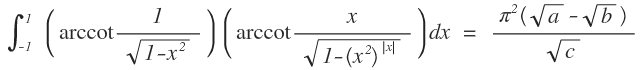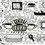# Isn't it easy$\large \int _{ -1 }^{ 1 }{ \left( \cot^{-1}{ \frac { 1 }{ \sqrt { 1-{ x }^{ 2 } } } } \right) } \left( \cot^{-1}{ \frac { x }{ \sqrt { 1-{ \left( { x }^{ 2 } \right) }^{ \left| x \right| } } } } \right) dx\quad =\quad \frac { { \pi }^{ 2 }\left( \sqrt { a } -\sqrt { b } \right) }{ \sqrt { c } }$

With $a,b,c$ are positive integers in their lowest form, find $a+b+c$.Note by Abhimanyu Swami
5 years, 7 months ago

This discussion board is a place to discuss our Daily Challenges and the math and science related to those challenges. Explanations are more than just a solution — they should explain the steps and thinking strategies that you used to obtain the solution. Comments should further the discussion of math and science.

When posting on Brilliant:

• Use the emojis to react to an explanation, whether you're congratulating a job well done , or just really confused .
• Ask specific questions about the challenge or the steps in somebody's explanation. Well-posed questions can add a lot to the discussion, but posting "I don't understand!" doesn't help anyone.
• Try to contribute something new to the discussion, whether it is an extension, generalization or other idea related to the challenge.

MarkdownAppears as
*italics* or _italics_ italics
**bold** or __bold__ bold
- bulleted- list
• bulleted
• list
1. numbered2. list
1. numbered
2. list
Note: you must add a full line of space before and after lists for them to show up correctly
paragraph 1paragraph 2

paragraph 1

paragraph 2

[example link](https://brilliant.org)example link
> This is a quote
This is a quote
    # I indented these lines
# 4 spaces, and now they show
# up as a code block.

print "hello world"
# I indented these lines
# 4 spaces, and now they show
# up as a code block.

print "hello world"
MathAppears as
Remember to wrap math in $$ ... $$ or $ ... $ to ensure proper formatting.
2 \times 3 $2 \times 3$
2^{34} $2^{34}$
a_{i-1} $a_{i-1}$
\frac{2}{3} $\frac{2}{3}$
\sqrt{2} $\sqrt{2}$
\sum_{i=1}^3 $\sum_{i=1}^3$
\sin \theta $\sin \theta$
\boxed{123} $\boxed{123}$

Sort by:

@Abhimanyu Swami Since it's really late, I'll just write part of the solution and will complete it sometime tomorrow.  Use the property $\int_a^b f(x)dx = \int_a^b f(a+b-x)dx$ Notice that we can thus write  $I=\int _{ -1 }^{ 1 }{ \left( \cot^{-1}{ \frac { 1 }{ \sqrt { 1-{ x }^{ 2 } } } } \right) } \left( \cot^{-1}{ \frac { x }{ \sqrt { 1-{ \left( { x }^{ 2 } \right) }^{ \left| x \right| } } } } \right) dx\quad$  $I=\int _{ -1 }^{ 1 }{ \left( \cot^{-1}{ \frac { 1 }{ \sqrt { 1-{ (-x) }^{ 2 } } } } \right) } \left( \cot^{-1}{ \frac { (-x) }{ \sqrt { 1-{ \left( { (-x) }^{ 2 } \right) }^{ \left| -x \right| } } } } \right) dx\quad$ Add both to get $\Longrightarrow I=\dfrac{1}{2}\int_{-1}^1 \dfrac{\pi^2}{2} - \pi \tan^{-1}\left(\dfrac{1}{\sqrt{1-x^2}}\right)dx$  The rest should then become easy.  Your final result seems to be $I = \dfrac{\pi^2}{2} \left(\sqrt{2}-1\right)$  Hope this helps.

- 5 years, 5 months ago

YEAH THANKS FOR THAT.

- 5 years, 5 months ago

Welcome! Glad to have been of help! On a side note, you shouldn't write everything in all caps since it's considered rude on the Net. I of course have no objection, but others might...

- 5 years, 5 months ago

Oh sorry , It wasn't intentional.

- 5 years, 5 months ago

Woah! Nice! I didn't know of that property.

- 5 years, 5 months ago

Thanks very much!

- 5 years, 5 months ago

In fact, this property is very useful when dealing with Inverse Trig Integrals, and is also a useful thing to check for when you see an integral and nothing strikes you about how to solve it (this experience of nothing striking you must be a rarity for you!).

- 5 years, 5 months ago

Nah! It's not rare. In fact, it happens all the time. But yeah really thanks for sharing this, I learned a new thing. Are there any more such properties? *This looks like a typical coaching institute property :P

- 5 years, 5 months ago

You're too modest! I wouldn't be able to tell unless you tell me the properties you already know. Actually, it's just that people rarely remember this property. Haha, I've only started going to a coaching institute this month and they haven't even taught limits!

- 5 years, 5 months ago

@Kartik Sharma Why don't you post some problems on Integration? We could all learn an enormous amount from you. By the way, try this-you'll hopefully like it:)

- 5 years, 5 months ago

which coaching are u referring to!? @Ishan Dasgupta Samarendra

- 5 years, 5 months ago

FIITJEE (South Delhi Center).

- 5 years, 5 months ago

u mean kalu sarai na!!

- 5 years, 5 months ago

Yeah!

- 5 years, 5 months ago

Well, yeah! So, you're in 11th only? I thought you are in 12th since you're 16.

- 5 years, 5 months ago

Yes, I'm in 11 only. Late admission... So when do we see your problems on Integration? Quite excited already!

- 5 years, 5 months ago